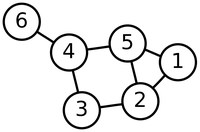# Glossary

## Edge

An edge connects two nodes of a graph with a segment or curve. If an edge connects $v$ to $w$, then we can denote the edge by $\{v, w\}$ or $\{w, v\}$; that is, the order in which the nodes are given in the edge does not matter. The graph below contains 7 edges, including $\{2, 3\}$ and $\{6, 4\}$.In directed graphs, the edges are provided with an orientation. As a result, the resulting directed edge is represented by an arrow connecting a tail node to a head node. If the edge connects $v$ to $w$, then we represent the directed edge by $(v, w)$ (but not by $(w, v)$).